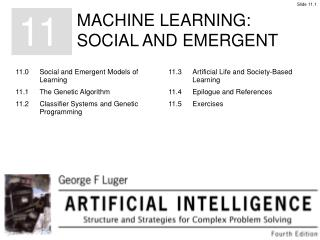Download PresentationA general form of the genetic algorithm:

# A general form of the genetic algorithm: - PowerPoint PPT PresentationDownload Presentation## A general form of the genetic algorithm:

- - - - - - - - - - - - - - - - - - - - - - - - - - - E N D - - - - - - - - - - - - - - - - - - - - - - - - - - -
##### Presentation Transcript

1. A general form of the genetic algorithm:

2. Figure 11.1:Use of crossover on two bit strings of length eight. # is “don’t care.”

3. Table 11.1:The gray coded bit patterns for the binary numbers 0, 1, . . ., 15.

4. Figure 11.2:Genetic algorithms visualized as parallel hill climbing,adapted from Holland (1986).

5. Figure 11.3:A classifier system interacting with the environment,adapted from Holland (1986).

6. Figure 11.4:The random generation of a program to initialize. Thecircled nodes are from the set of functions.

7. Figure 11.5:Two programs, selected on fitness for crossover. Point | froma and b are randomly selected for crossover.

8. Figure 11.6:The child programs produced by crossover of the points in Figure 11.5.

9. Table 11.3:A set of fitness cases, with planetary data taken from Urey (1952). A is Earth’s semi-major axis of orbit and P is in units of earth-years.

10. Figure 11.7:The target program relating orbit to period for Kepler’sThird Law.

11. Figure 11.8:Members from the initial population of programs to solvethe orbital period problem.

12. Figure 11.9:The shaded region indicates the set of neighbors for the “game of life.”

13. Figure 11.10:A set of neighbors generating the “blinking” light phenomenon. Figure 11.11:What happens to these patterns at the next time cycle?

14. Figure 11.12:A “glider” moves across the display. Figure 11.13:A “glider” is “consumed” by another “entity.”

15. Figure 11.14: Space-time diagrams showing the behavior of two As, discovered by the genetic algorithm on different runs. They employ embedded particles for the non local computation or general emerging patterns seen. Each space-time diagram iterates over a range of time steps, with 1s given as black cells, 0s as white cells; time increases down the page, from Crutchfield and Mitchell (1994).

16. Figure 11.15: Illustration of a one-dimensional, binary-state, nearest-neighbor cellular automaton with N = 11. Both the lattice and the rule table for updating the lattice are illustrated. The lattice configuration is shown over one time step. The cellular automaton is circular in that the two end values are neighbors.

17. Table 11.4: Catalog of regular domains, particles (domain boundaries), particle velocities (in parentheses), and particle interactions of the space-time behavior of the CA of Figure 11.14a. The notation p ~ xy means that p is the particle forming the boundary between regular domains x and y.

18. Figure 11.16: Analysis of the emergent logic for density classification of 11.14a.This CA has three domains, six particles, and six particle iterations, as noted in Table 11.3. The domains have been filtered out using an18-state nonlinear transducer; adapted from Crutchfield and Mitchell (1994).